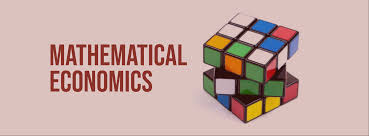#### BA Econ 2B - Mathematical Economics (22-1)

Mathematical Economics is a 3-unit basic core course subject offered to BA Economics students. This is designed to give the students the knowledge of the different mathematical techniques in the context of economics application that includes Algebra topics (i.e. algebraic expressions, graphs and functions, linear equations, quadratic equations), matrices, differentiation, and financial mathematics. The students will determine and calculate different economics concepts through Algebra computations, apply appropriate mathematical methods to solve problems involving the growth and decline over discrete time periods of some economic variables, solve a system of simultaneous equations through the use of matrices both manually and using a spreadsheet, and derive different economic concepts using differentiation. The students are encouraged to write a data analysis paper integrating the different mathematical concepts and techniques in an economics context.